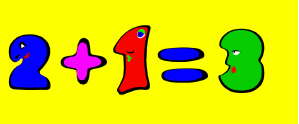Addition and subtraction are the simplest and most basic mathematical operations. Addition combines two or several quantities into a larger quantity (Adding – sign: +), while during subtraction, a number of objects is subtracted from a different number of objects (Subtracting – sign: -). Subtraction is the inverse operation of addition. It has two important property: commutativity and associativity. Commutativity means that the order of elements does not influence the result – you can switch places of the objects you want add and the result will be the same. Associativity means that the order in which you perform adding (when you are trying to add more than two numbers) is not important. For example:

a + (b + c) = (a + b) + c

Another important thing to remember is that adding zero to any other number does not change that number. Five + zero is always five and that is true for all other numbers when zero is added to them.

As for subtraction, it does not share the same properties as addition. When you subtract, the order of elements is very important. As you already know well, five minus three is not the same as three minus five. Zero is neutral in this operation as well – if zero is subtracted from any other number, that number does not change.